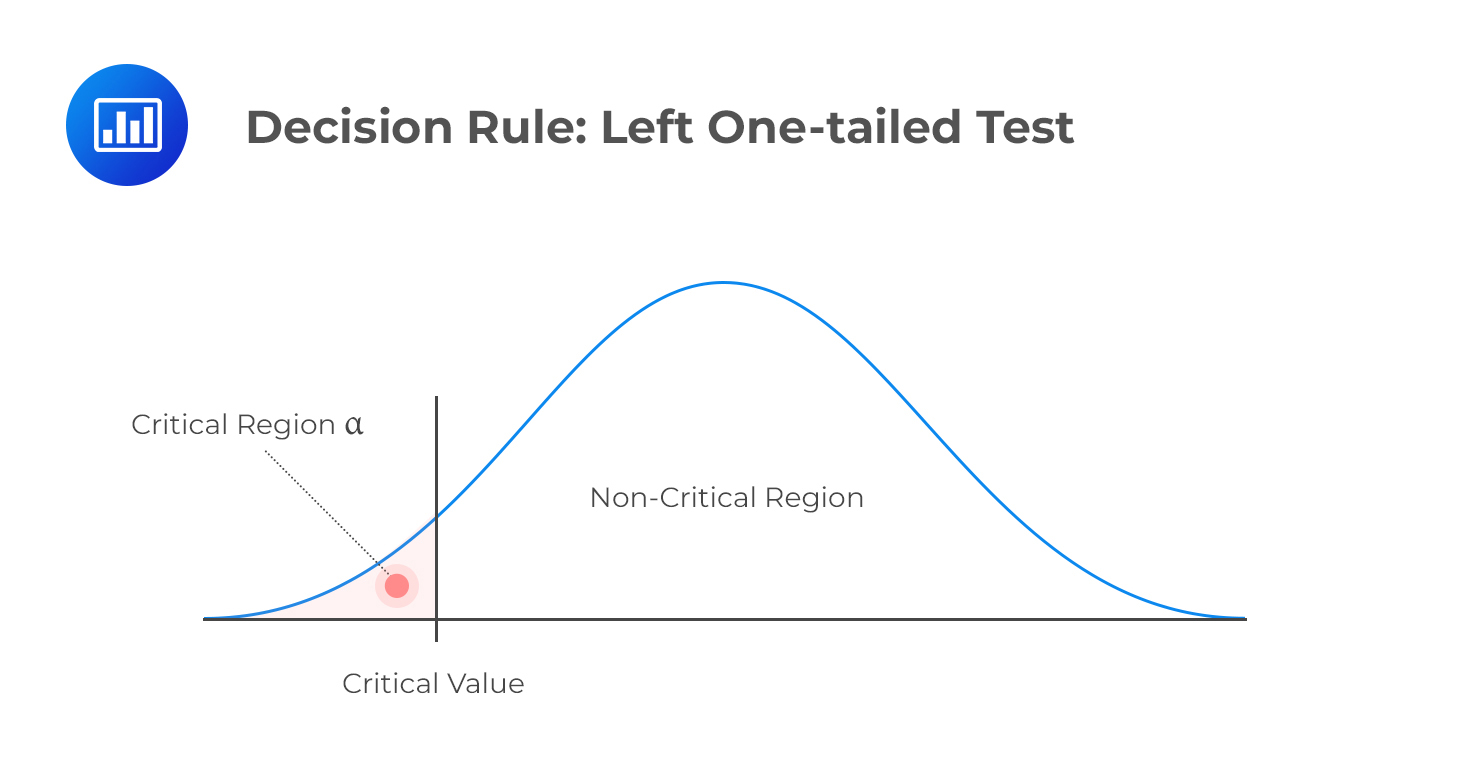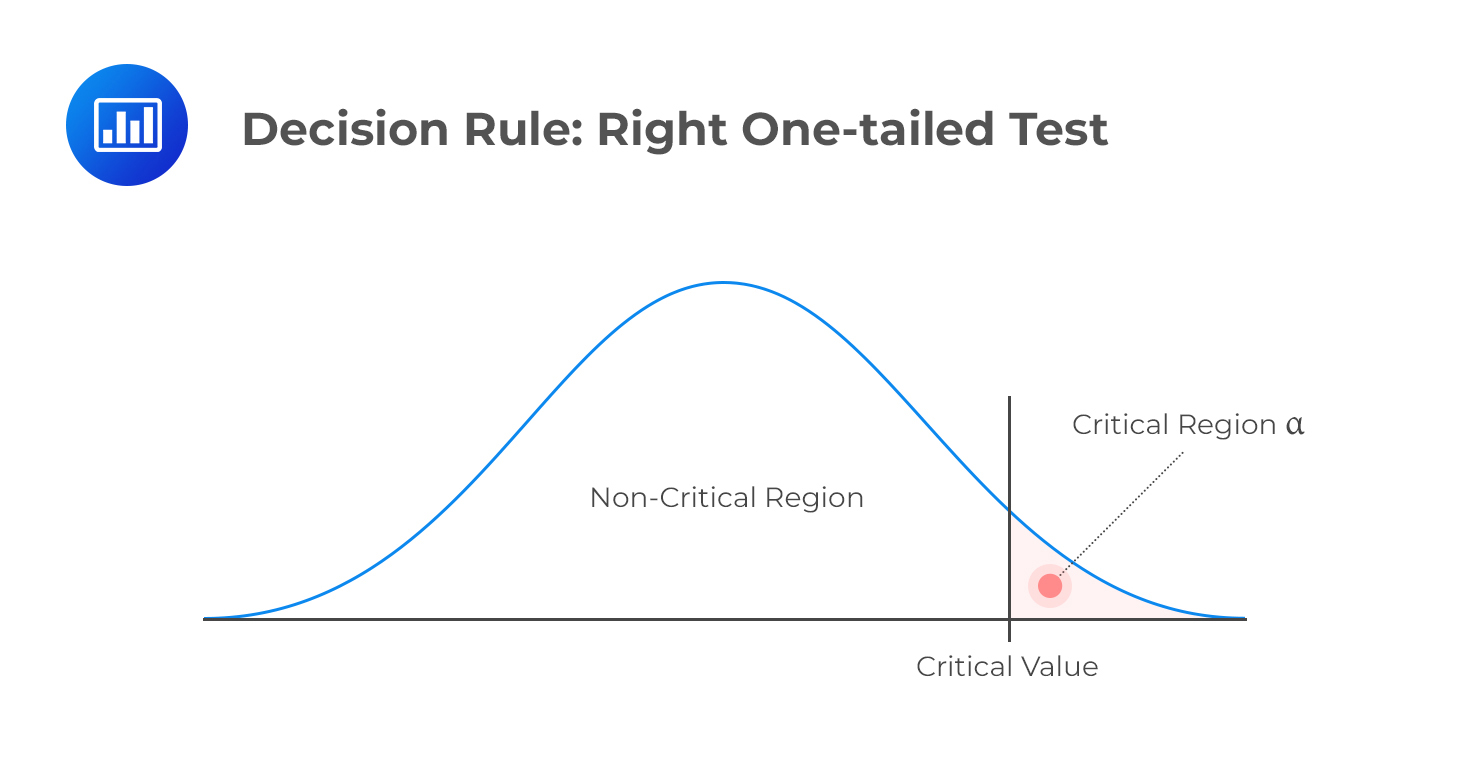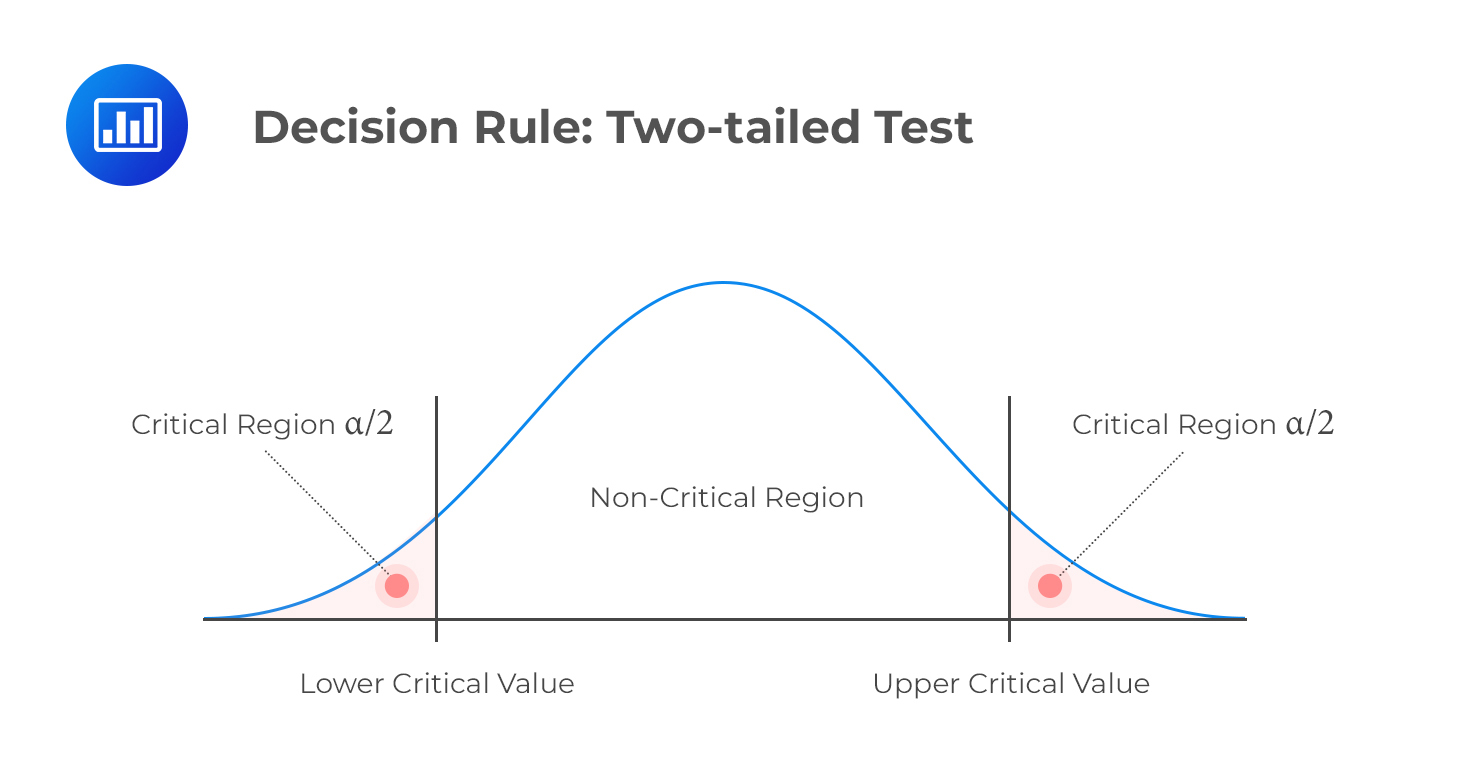Limited Time Offer: Save 10% on all 2022 Premium Study Packages with promo code: BLOG10# Understanding the Decision Rule

The decision rule refers to the procedure followed by analysts and researchers when deciding whether to reject or not to reject a null hypothesis. We use the phrase “not to reject” because it is considered statistically incorrect to “accept” a null hypothesis. Rather, we can only assemble enough evidence to support it.

## Breaking Down the Decision Rule

The decision to reject or not reject a null hypothesis is based on the distribution the test statistic assumes. This means that if the variable involved follows a normal distribution, we use the level of significance of the test to come up with critical values that lie along the standard normal distribution.

Note that prior to decision making, one must consider whether the test should be one-tailed or two-tailed. This is because the number of tails determines the value of α (significance level). The following is a summary of the decision rules under different scenarios.

### Left One-tailed Test

H1: parameter < X

Decision rule: reject H0 if the test statistic is less than the critical value. Otherwise, do not reject H0.### Right One-tailed Test

H1: parameter > X

Decision rule: reject H0 if the test statistic is greater than the critical value. Otherwise, do not reject H0.### Two-tailed Test

H1: parameter X (not equal to X)

Decision rule: reject H0 if the test statistic is greater than the upper critical value or less than the lower critical value.## The Power of a Test

The power of a test is the direct opposite of the level of significance. While the level of significance gives us the probability of rejecting a null hypothesis when it is, in fact, true, the power of a test gives the probability of correctly discrediting and rejecting a null hypothesis when it is false. In other words, it gives the likelihood of rejecting a H0 when indeed, it is false. Expressed mathematically,

$$\text{Power of a test} = 1 – P(\text{type } II \text{ error})$$

When presented with a situation where there are multiple test results for the same purpose, it is the test with the highest power that is considered the best.

## The Link Between Confidence Interval and Hypothesis Testing

Critical values link confidence intervals and hypothesis tests. For example, to construct a 95% confidence interval assuming a normal distribution, we would need to determine the critical values that correspond to a 5% significance level. Similarly, if we were to conduct a test of some given hypothesis at the 5% significance level, we would use the same critical values used for the confidence interval to subdivide the distribution space into rejection and non-rejection regions.

### Example: Hypothesis Testing

A survey carried out using a sample of 50 CFA Level I candidates reveals an average IQ of 105. Assuming that IQs are distributed normally, carry out a statistical test to determine whether the mean IQ is greater than 100. You are instructed to use a 5% level of significance. (Previous studies give a standard deviation of IQs of approximately 20.)

Solution

First, state the hypothesis:

H0: μ = 100 vs H1: μ > 100

Since IQs follow a normal distribution, under $$H_0, \frac {(X’ – 100)}{\left( \frac {\mu}{\sqrt n} \right)} \sim N(0,1)$$

Next, we compute the test statistic, which is $$\frac {(105 – 100)}{\left(\frac {20}{\sqrt {50}} \right)} = 1.768$$

This is a right one-tailed test, and IQs are distributed normally. Therefore, we should compare our test statistic to the upper 5% point of the normal distribution.

From the normal distribution table, this value is 1.6449. Since 1.768 is greater than 1.6449, we have sufficient evidence to reject the H0 at the 5% significance level. Therefore, it is reasonable to conclude that the mean IQ of CFA candidates is greater than 100.

(Note the choice of words used in the decision-making part and the conclusion.)

## Question

Use data from the previous example to carry out a test at 5% significance to determine whether the average IQ of candidates is greater than 102.

1. There is sufficient evidence to reject the H0 and conclude that the average IQ is greater than 102.
2. There is insufficient evidence to reject the H0 and therefore it is reasonable to conclude that the average IQ is not more than 102.
3. There is sufficient evidence to reject the H0 and therefore it is reasonable to conclude that the average IQ is greater than 102.

Solution

The correct answer is B.

Just like in the example above, start with stating the hypothesis;

H0: μ = 100 vs. H1: μ > 102

The test statistic is $$\frac {(105 – 102)}{\left( \frac {20}{\sqrt{50}} \right)} = 1.061$$

Again, this is a right one-tailed test. 1.061 is less than the upper 5% point of a standard normal distribution (1.6449). Therefore, we do not have sufficient evidence to reject the H0 at the 5% level of significance. It is, therefore, reasonable to conclude that the average IQ of CFA candidates is not more than 102.

Shop CFA® Exam Prep

Offered by AnalystPrepLevel I
Level II
Level III
All Three Levels
Featured Shop FRM® Exam PrepFRM Part I
FRM Part II
FRM Part I & Part II
Learn with Us

Subscribe to our newsletter and keep up with the latest and greatest tips for success
Shop Actuarial Exams PrepExam P (Probability)
Exam FM (Financial Mathematics)
Exams P & FM
Shop GMAT® Exam PrepComplete CourseSergio Torrico
2021-07-23
Excelente para el FRM 2 Escribo esta revisión en español para los hispanohablantes, soy de Bolivia, y utilicé AnalystPrep para dudas y consultas sobre mi preparación para el FRM nivel 2 (lo tomé una sola vez y aprobé muy bien), siempre tuve un soporte claro, directo y rápido, el material sale rápido cuando hay cambios en el temario de GARP, y los ejercicios y exámenes son muy útiles para practicar.diana
2021-07-17
So helpful. I have been using the videos to prepare for the CFA Level II exam. The videos signpost the reading contents, explain the concepts and provide additional context for specific concepts. The fun light-hearted analogies are also a welcome break to some very dry content. I usually watch the videos before going into more in-depth reading and they are a good way to avoid being overwhelmed by the sheer volume of content when you look at the readings.Kriti Dhawan
2021-07-16
A great curriculum provider. James sir explains the concept so well that rather than memorising it, you tend to intuitively understand and absorb them. Thank you ! Grateful I saw this at the right time for my CFA prep.nikhil kumar
2021-06-28
Very well explained and gives a great insight about topics in a very short time. Glad to have found Professor Forjan's lectures.Marwan
2021-06-22
Great support throughout the course by the team, did not feel neglectedBenjamin anonymous
2021-05-10
I loved using AnalystPrep for FRM. QBank is huge, videos are great. Would recommend to a friendDaniel Glyn
2021-03-24
I have finished my FRM1 thanks to AnalystPrep. And now using AnalystPrep for my FRM2 preparation. Professor Forjan is brilliant. He gives such good explanations and analogies. And more than anything makes learning fun. A big thank you to Analystprep and Professor Forjan. 5 stars all the way!michael walshe
2021-03-18
Professor James' videos are excellent for understanding the underlying theories behind financial engineering / financial analysis. The AnalystPrep videos were better than any of the others that I searched through on YouTube for providing a clear explanation of some concepts, such as Portfolio theory, CAPM, and Arbitrage Pricing theory. Watching these cleared up many of the unclarities I had in my head. Highly recommended.